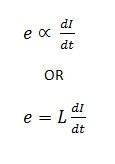# Self Induced EMF

Definition: Self-induced emf is the e.m.f induced in the coil due to the change of flux produced by linking it with its own turns. This phenomenon of self-induced emf can be further understood by the following example given below:Consider a coil having N number of turns as shown in the above figure. When the switch S is closed and current I flows through the coil, it produces flux (φ) linking with its own turns. If the current flowing through the coil is changed by changing the value of variable resistance (R), the flux linking with it, changes and hence emf is induced in the coil. This induced emf is called Self Induced emf.

The direction of this induced emf is such that it opposes its very own cause which produces it, that means it opposes the change of current in the coil. This effect is because of Lenz’s Law.

Since the rate of change of flux linking with the coil depends upon the rate of current in the coil.The magnitude of self-induced emf is directly proportional to the rate of change of current in the coil. L is constant of proportionality and called as Self Inductance or the Coefficient of Self Inductance or Inductance of the coil.

### 1 thought on “Self Induced EMF”

1. Very nice site congratulations u have all the information about the diploma and semesters subject Basic electronic,Elements of Electrical Engineering. I will suggest your site to my friends☺️☺️☺️☺️☺️👍👍👍👍😊😊😊😊😊💐💐💐💐💐👌👌👌👌👌👌👌